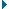# 捕获按键

```Key.getCode();
```

• 要获得上次所按键的虚拟键控代码，可使用 `getCode()` 方法。
• 要获得上次所按键的 ASCII 值，可使用 `getAscii()` 方法。

ASCII 值分配给每个字符集的前 127 个字符。ASCII 值提供了有关屏幕上字符的信息。例如，字母“A”和字母“a”有不同的 ASCII 值。

• 查看键盘键和键控代码值中的键控代码列表。
• 使用 Key 类常数。（在“动作”工具箱中单击“内置类”类别，然后单击“Movie”，之后单击“Key”，最后单击“Constants”。）
• 将以下 `onClipEvent()` 处理函数分配给影片剪辑，然后选择“控制”>“测试影片”并按所需键。
```onClipEvent(keyDown) {
trace(Key.getCode());
}
```

####创建一个能被键盘激活的影片剪辑：

1. 在舞台上创建一个影片剪辑，该影片剪辑将会随键盘箭头的活动而移动。

在此示例中，影片剪辑实例名称为 `car`

2. 在舞台上创建一个随汽车方向更新的动态文本框。使用属性检查器，给它指定以下实例名：`display_txt`

注意：不要把变量名和实例名混淆。有关更多信息，请参见关于文本字段实例和变量名称

3. 在时间轴上选择第 1 帧；如果看不到“动作”面板，则选择“窗口”>“开发面板”>“动作”将其打开。
4. 要设置每次按键时汽车沿屏幕移动的距离，请定义 `distance` 变量并将它的初始值设置为 10。
```var distance = 10;
```
5. 要为汽车影片剪辑创建事件处理函数，以检查当前按下的是哪一个箭头键（向左、向右、向上或向下），请向“动作”面板添加以下代码：
```car.onEnterFrame = function() {

}
```
6. 将一个 `with` 语句添加到 `onEnterFrame` 处理函数的主体中，并将 `car` 指定为 `with` 语句的
对象。

您的代码应该如下所示：

```var distance = 10;
car.onEnterFrame = function() {
with (car) {
}
}
```
7. 若要检查是否已按下右箭头键，并相应地移动汽车影片剪辑，请将以下代码添加到 `with` 语句的主体中。您的代码应该如下所示：
```distance = 10;
car.onEnterFrame = function() {
with (car) {
if (Key.isDown(Key.RIGHT)) {
_x += distance;
if (_x >= 400) {
_x = 400;
}
_root.display_txt.text = "Right";
}
}
}
```

如果按下了右箭头键，则汽车的 `_x` 属性将按 `distance` 变量指定的量增加值。下一个 `if` 语句测试剪辑的 `_x` 属性的值是否大于或等于 400 (`if(_x >=400)`)；如果是，则其位置将固定在 400。另外，Right 一词应显示在 SWF 文件中。

8. 使用类似的代码检查是否按下了左箭头键、上箭头键或下箭头键。您的代码应该如下所示：
```var distance = 10;
car.onEnterFrame = function() {
with (car) {
if (Key.isDown(Key.RIGHT)) {
_x += distance;
if (_x >= 400) {
_x = 400;
}
_root.display_txt.text = "Right";
} else if (Key.isDown(Key.LEFT)) {
_x -= distance;
if (_x < 0) {
_x = 0;
}
_root.display_txt.text = "Left";
} else if (Key.isDown(Key.UP)) {
_y -= distance;
if (_y < 0) {
_y =   0 ;
}
_root.display_txt.text = "Up";
} else if (Key.isDown(Key.DOWN)) {
_y += distance;
if (_y > 300) {
_y = 300;
}
_root.display_txt.text = "Down";
}
}
}
```
9. 选择“控制”>“测试影片”对文件进行测试。